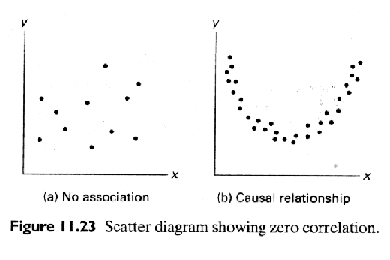Section 11.5 Inferences Concerning the Regression Coefficients

Example 11.2

Confidence Interval for b , the slope parameter

A (1-a)100% confidence interval for the parameter b in the regression line mY|x  =  a + bx  is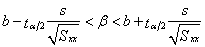whereis a value of the t-distribution with n-2 degrees of freedom

## Example 11.2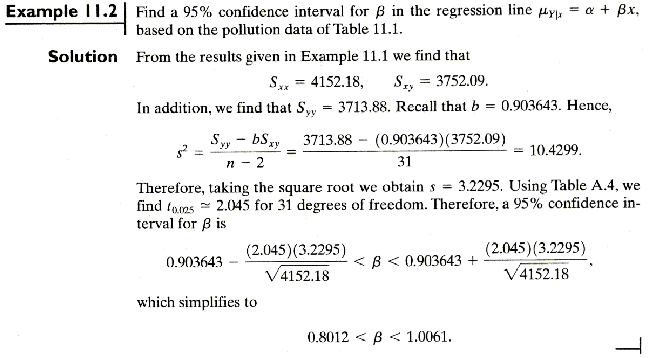Hypothesis testing on the slope parameter, b

To test the null hypothesis Ho:b = bo against any suitable alternatives,

use t-distribution with n-2 degrees of freedom to define the critical region, and the following test statistic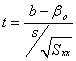## Example 11.3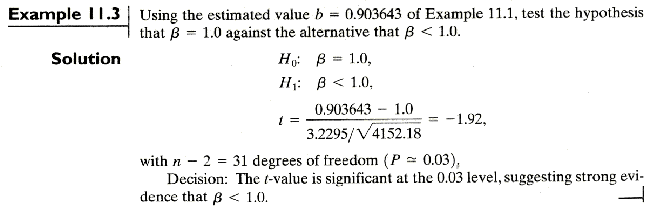## Minitab Example: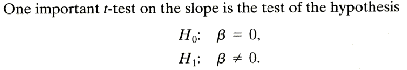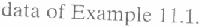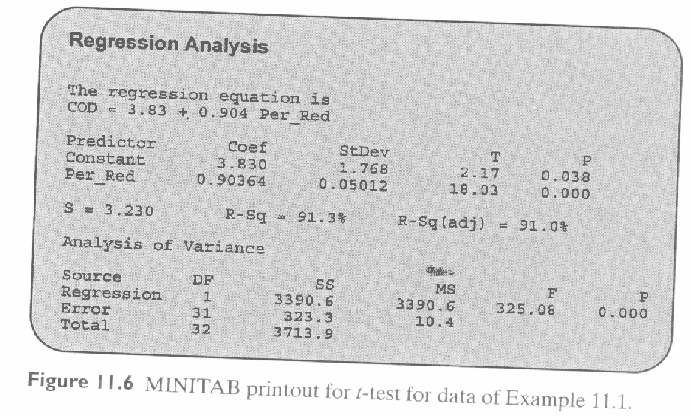Statistical Inference on the intercept

Confidence Interval for a , the intercept parameter

A (1-a)100% confidence interval for the parameter a  in the regression line mY|x  =  a + bx  isor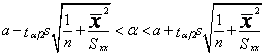whereis a value of the t-distribution with n-2 degrees of freedom

## Example 11.4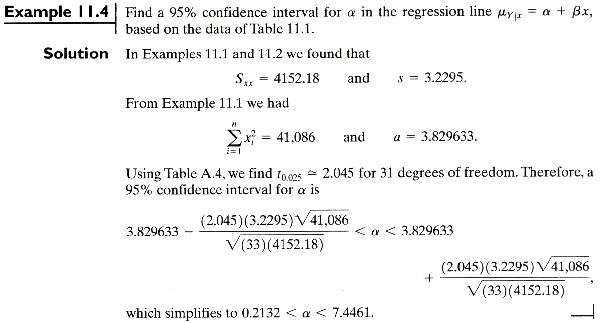Hypothesis testing on the intercept parameter, a

To test the null hypothesis Ho: a = ao against any suitable alternatives,

use t-distribution with n-2 degrees of freedom to define the critical region, and the following test statistic## Example 11.5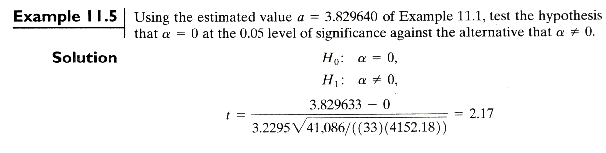df=33-2=31, P-value =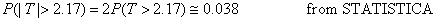From Table A.4 directly, P-value < 0.05. So, the null hypothesis of zero intercept is rejected at 0.05 level of significance.

A measure of quality of fit: Coefficient of Determination, R2

Coefficient of Determination, R2 = proportion of total variability in the dependent variable Y explained by the fitted model.

TSS = SSR + SSE

Coefficient of Determination,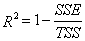R2 = 1.0                 if fit is perfect

R2 = 0.0                 if fit is poor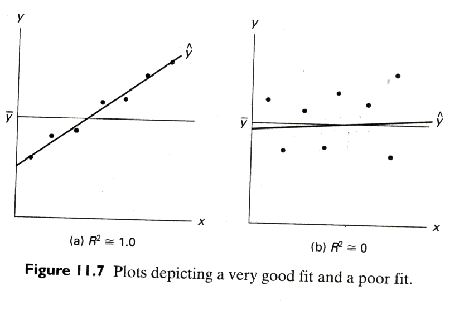Section 11.6 Prediction

Confidence Interval for, the mean response

A (1-a)100% confidence interval for the mean responsein the regression line mY|x  =  a + bx  is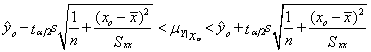whereis a value of the t-distribution with n-2 degrees of freedom

## Example 11.6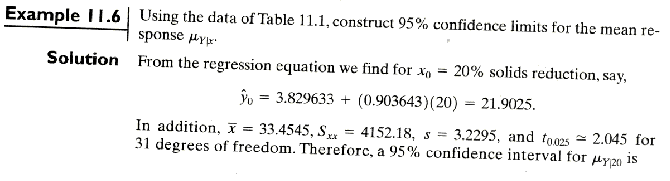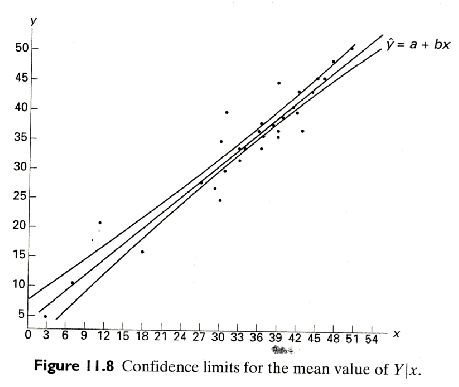Prediction Interval for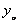, the future value of a response

A (1-a)100% prediction interval for a single responsein the regression line mY|x  =  a + bx  is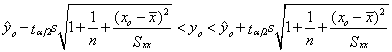whereis a value of the t-distribution with n-2 degrees of freedom

## Example 11.7Section 11.11  Simple Linear Regression Case Study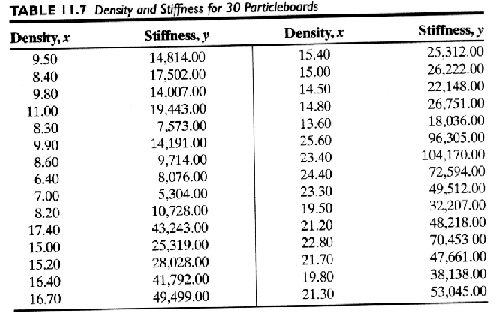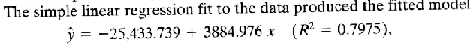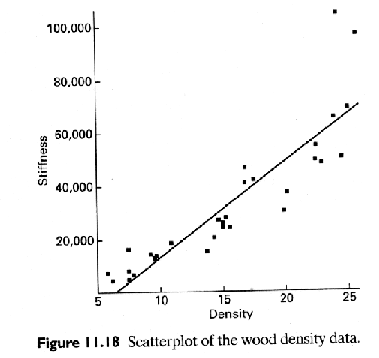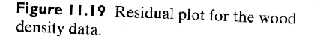A more complicated model may be more appropriate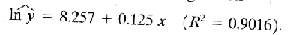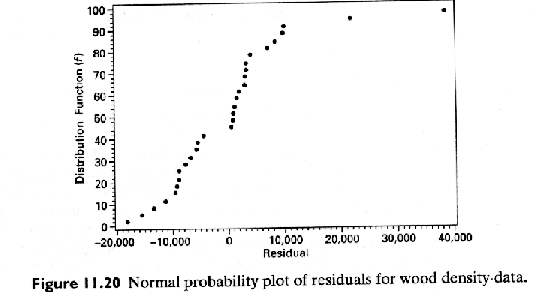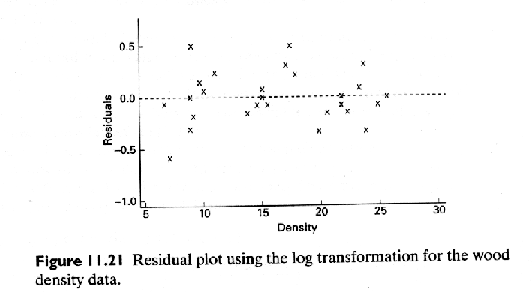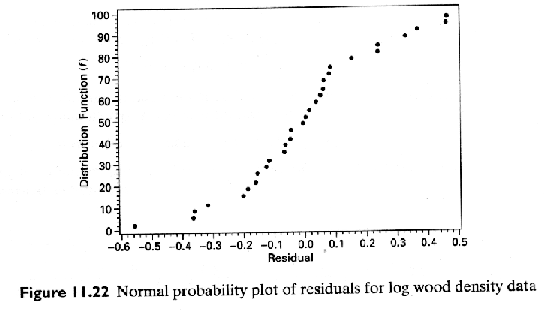The higher R2 value would suggest that the transformed model is more appropriate.

Section 11.12 Correlation

Correlation coefficient

The measure r of linear association between two variables X and Y is estimated by the sample correlation coefficient r, where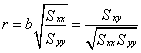-1 <  r < 1

r = 1.0 or –1.0       perfect linear relationship

r = 0.0                   no linear relationship

Sample coefficient of determination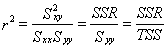represents the proportion of the variation in TSS explained by the regression of Y on x, namely, SSR.

## Example 11.10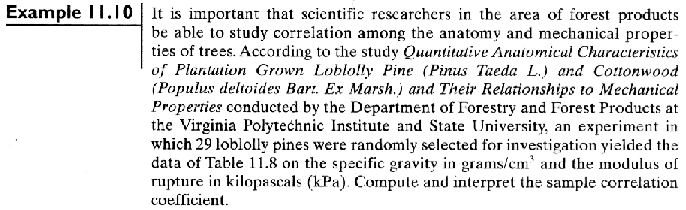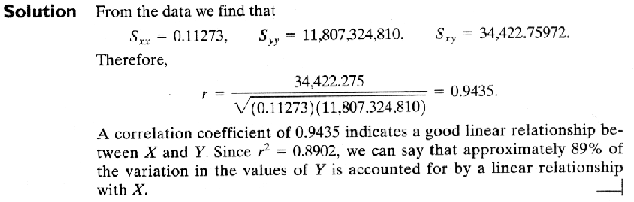Hypothesis testing on the correlation coefficient, r

To test the null hypothesis Ho: r = ro against any suitable alternatives,

We can use t-distribution with n-2 degrees of freedom to define the critical region, and the following test statisticbut this test has problem when r values close to -1 or 1

However, a more general test is given by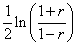the approximate normal distribution with mean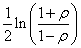and variance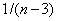.

We can use the standard normal to define the critical region, and the following test statistic## Example 11.11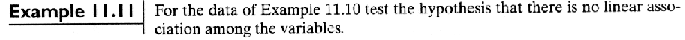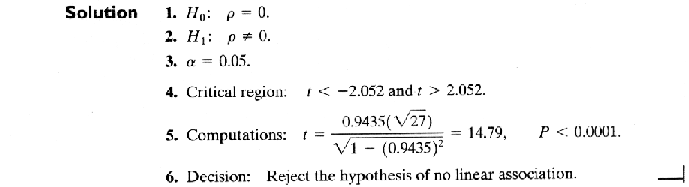## Example 11.12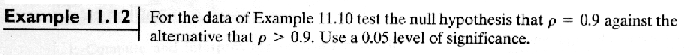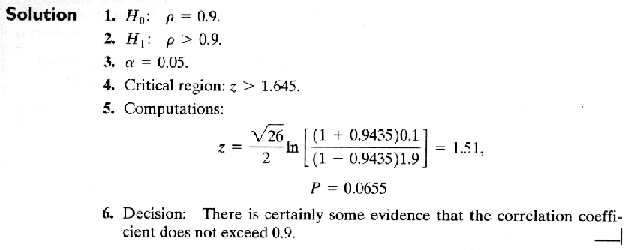Important: Correlation is a measure of linear relationship, so r = 0 does not necessarily mean there is no relationship between two variables.